### 13.1.2.3 A simple unicycle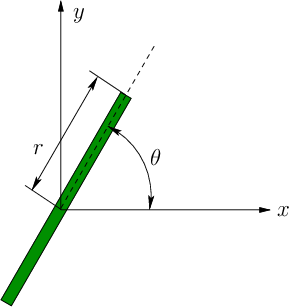Consider the simple model of a unicycle, which is shown in Figure 13.5. Ignoring balancing concerns, there are two action variables. The rider of the unicycle can set the pedaling speed and the orientation of the wheel with respect to the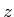-axis. Let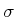denote the pedaling angular velocity, and let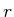be the wheel radius. The speed of the unicycle is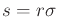. In this model, the speed is set directly by an action variable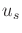(alternatively, the pedaling rate could be an action variable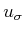, and the speed is derived as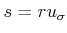). Let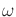be the angular velocity of the unicycle orientation in the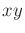plane (hence,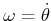). Letbe directly set by an action variable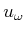. The configuration transition equation is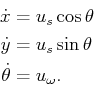(13.18)

This is just the differential drive equation (13.17) with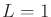and the substitution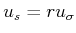. Thus, a differential drive can simulate a unicycle. This may seem strange; however, it is possible because these models do not consider dynamics. Note that the unicycle can also simulate the simple-car model. Therefore, the tricycle and unicycle models are similar.

Steven M LaValle 2020-08-14